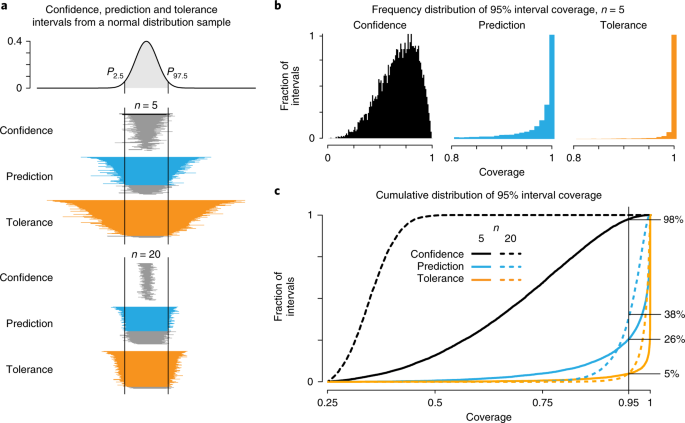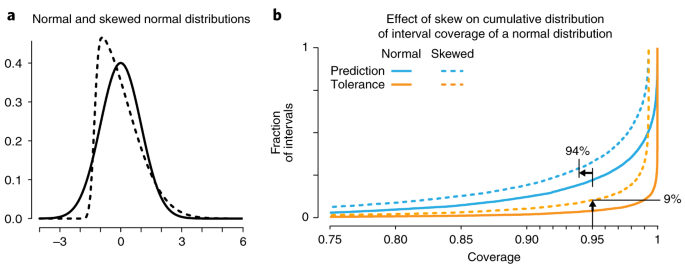POINTS OF SIGNIFICANCE

# Predicting with confidence and tolerance

I abhor averages. I like the individual case. –J.D. Brandeis.

Throughout our discussions, the process of assessing the plausible range for a population parameter has been a central theme. For example, the uncertainty in the population mean can be quantified with error bars based on standard error1, of which confidence intervals are one type. However, at times we may be interested less in the population parameters and more in typical values for individual samples drawn from the population. For example, if the mean height for 12-year-old boys in the United States is 149 cm, how unusual is it for a boy’s height to be outside the range of 145–155 cm? Much like for error bars, there are technical details in the answer to this question that often lead to confusion.

When the entire population has been observed, the central proportion p of the distribution (e.g., 95%) can be determined from the corresponding lower and upper (1 – p)/2 population percentiles (e.g., 2.5% and 97.5%). Alternatively, if we were interested only in the lower 95% of the population, we would use everything below the 95th percentile. For brevity, we will use Pk (e.g., P95) here to indicate percentiles.

Because we almost never have access to the entire population, we must estimate percentiles from samples. The simplest way of estimating the Pk percentile from a sample of size n is to use the knth value of the ordered sample, and interpolate between adjacent values if this is not an integer. However, this approach does not use all the information in the sample, and a large sample is required when k is very small (or large). For example, we cannot distinguish between P90 and P95 for n = 10, because the method returns the largest sample value for both.

In Fig. 1 we show distributions of 1,000 P95 estimates of a standard normal distribution determined via the interpolation method for sample sizes n = 25, 50, 100 and 250. For n = 50, the mean of the sampling distribution is 1.54, which is systematically 6% lower than the actual value of 1.64; this bias can be mitigated by the use of larger samples or more complex estimation approaches. We can estimate our precision from the s.d. of the sampling distribution of P95. It is relatively large (s.d. = 0.27) and tells us that we can still obtain inaccurate estimates—for example, 15% of our P95 estimates are lower than P90 = 1.28, and 7% are higher than P97.5 = 1.96.Fig. 1: Precision and accuracy of the estimation of percentiles from samples.

Thus, two issues arise from our sample-based method. First, given that in many situations we cannot expect such large sample sizes, how can we estimate large (or small) percentiles? Second, how can we control the precision of these estimates?

For normally distributed data with mean μ and s.d. σ, an obvious approach is to use the fact that the central proportion p of the distribution is in the region of μ ± z(1+p)/2σ, where zk is the kth percentile of a standard normal2. For example, for p = 95%, (1 + p)/2 = 97.5 and z97.5 = 1.96. We are using σ, not the s.e.m., because we are estimating the spread of the population and not the uncertainty in estimating the mean.

Unfortunately, this approach does not account for the uncertainty in estimating μ and σ on the basis of the sample mean, $$\bar{x}$$, and sample s.d., s. Even if we actually knew σ but not μ, we would still need to consider that the expected squared difference between a randomly selected element of the population and the sample mean is not σ2 but rather σ2 + σ2/n. The second term is the squared s.e.m., which accounts for the uncertainty in the sample mean. We can account for the variability of the sample s.d. by using the quantiles of the Student’s t distribution, which mitigates the problem of underestimation of σ with small samples2. By applying both corrections, we obtain the prediction interval $$\bar{x}\pm t_{n-1,(1+p)/2}sc$$, where c = √(1 + 1/n) and tn–1,(1+p)/2 is the (1 + p)/2 percentile of the t distribution on d.f. = n – 1, the same as for a one-sample t-test with the same sample size.

Prediction intervals are straightforward to compute, but their interpretation can give rise to confusion. The p prediction interval is an interval that, on average, covers percentage p of the population. This statement is equivalent to saying that, on average, the percentage p of new values drawn from the population will fall in the interval. For example, if we calculated many p = 95% prediction intervals, we would find that their average coverage was 95%, but that there was a distribution of coverages, with some intervals having less than 95% coverage. For a given prediction interval, we have no confidence of its coverage.

The combination of prediction and confidence is incorporated in a third kind of interval, the tolerance interval, which is defined by two values, p and α (ref. 3). The first parameter, p, works just like prediction intervals, and the second, α, importantly controls the probability that the coverage is at least p. If we calculated many p tolerance intervals, we would find that the fraction 1 – α had a coverage of p or more (thus their average coverage would be considerably higher than p).

The tolerance interval has the same form as a confidence or prediction interval, $$\bar{x} \pm sC$$, but here C takes into account all of the uncertainty in the estimation—the selection of the sample, as well as the estimation of the mean and of the s.d. For normal distributions, an exact value for C can be calculated. A commonly used approximation is C = z(1+p)/2c√(ν/X1–α,ν), where c = √(1 + 1/n), ν is the d.f. associated with estimation of the s.d., and X1–α,ν is the αth percentile of the χ2 distribution on ν d.f.

Figure 2a compares the sizes of 100 95% confidence, 95% prediction and tolerance intervals (p = 0.95, α = 0.05) from normally distributed samples of sizes n = 5 and n = 20. The confidence intervals are the shortest, as expected, as they measure the uncertainty in determining the mean of the distribution, not of a new sample. Only a small fraction of these intervals (8%) cover more than 95% of the distribution, and the average coverage of the intervals is 68%.Fig. 2: Size and coverage of confidence, prediction and tolerance intervals for a normal distribution determined from samples.

The prediction intervals are much larger (Fig. 2a, blue) than the confidence intervals. Their average coverage is (by definition) 95%, but a considerable number of intervals have less coverage, reflected in the long left tail of their coverage distribution (Fig. 2b, blue). For n = 5 we find that 26% of these intervals have coverage of less than 95%, and this value increases to 38% for n = 20 (Fig. 2c). To control this fraction, we would set (for example) α = 0.05 and use tolerance intervals, much in the same way as we would guard against false positives by applying a P value cutoff of P < α in a statistical test.

Tolerance intervals are larger than prediction intervals (Fig. 2a, orange), and their coverage distribution drops off much faster (Fig. 2b,c, orange). We find that 4.9% of these intervals have coverage of less than 95% in Fig. 2c, and, as expected, this value is not affected by sample size.

When the sample size increases, the sizes of the tolerance and prediction intervals become more similar, but their coverage requirements hold (Fig. 2a). These intervals do not shrink in the way that the confidence intervals do for larger samples (Fig. 2c) because they reflect the population spread.

Confidence intervals provide coverage of a single point—the population mean—with assurance that the probability of noncoverage is α. For example, a 1 – α confidence interval computed from a random sample of heights for 12-year-old boys should include the true mean in the 1 – α proportion of samples. However, this interval does not tell us about the typical height values in the sample.

In contrast, prediction and tolerance intervals both give information about typical values from the population and the percentage of the population expected to be in the interval. Tolerance intervals are constructed so that the interval covers the targeted percentage of the population with confidence 1 – α and are the preferred interval for this purpose. Prediction intervals are used more often and have the correct average coverage, but they provide no assurance of the probability of coverage. For example, for n = 5 and n = 20, our simulation shows that 26% and 38% of samples, respectively, may provide intervals with coverage that is too low (Fig. 2c).

There is a very strong caveat for prediction and tolerance intervals based on the normal distribution: unlike confidence intervals for the mean, they depend strongly on the normality of the data. For moderate to large samples, confidence intervals for the mean (and other population parameters such as variance and regression coefficients) do not require that the data be normal in order to be valid. This is because the central limit theorem guarantees that the sampling distribution of the estimate approaches normal as the sample size increases.

However, prediction and tolerance intervals are statements about the population—or can be thought of as statements about samples of size n = 1 (i.e., the comparison of the next sampled value against the interval). If the population is not close to normal, then the intervals can be inaccurate. For this reason, it is particularly important to pay attention to the normality assumption.

We illustrate this in Fig. 3a with normal and skewed normal distributions, both with mean = 0 and s.d. = 1. If we draw 10,000 samples of n = 5 from the skewed normal distribution under the assumption that they are from a normal distribution and calculate the cumulative coverage distributions as in Fig. 2c, we will find that the prediction intervals have slightly smaller average coverage (94%). The average coverage of tolerance intervals is still high (97%) but, importantly, their confidence is affected substantially: now 9% (nearly double the expected value) have less than 95% coverage (Fig. 3b; note that the horizontal scale is expanded relative to that in Fig. 2c). For a very skewed distribution, such as the exponential, our simulation yields an average coverage of 92% for prediction intervals, but now 19% of tolerance intervals have coverage less than 95%, even though their average coverage is still >95%.Fig. 3: The effect of departure from an assumption of normality on interval coverage.

Transformations such as logarithm and square root can be used with skewed non-negative data to put the data on a scale that is closer to the normal distribution. Alternatively, some software packages4 will compute prediction and tolerance intervals for some of the often-used distributions such as normal, Poisson and others.

Prediction and tolerance intervals are the obvious choice of intervals when the objective is to indicate a region of the original population in which unobserved values are expected to fall. Most observations are ‘typical’, not ‘average’, and these intervals should be used when observations are being labeled as unusual. For prediction with confidence, tolerance intervals are the most suitable.

## References

1. 1.

Krzywinski, M. & Altman, N. Nat. Methods 10, 921–922 (2013).

2. 2.

Krzywinski, M. & Altman, N. Nat. Methods 10, 1041–1042 (2013).

3. 3.

Howe, W. G. J. Am. Stat. Assoc. 64, 610–620 (1969).

4. 4.

Young, D. S. J. Stat. Softw. 36, 1–39 (2010).

## Author information

Authors

### Corresponding author

Correspondence to Martin Krzywinski.

## Ethics declarations

### Competing interests

The authors declare no competing interests.

## Rights and permissions

Reprints and Permissions